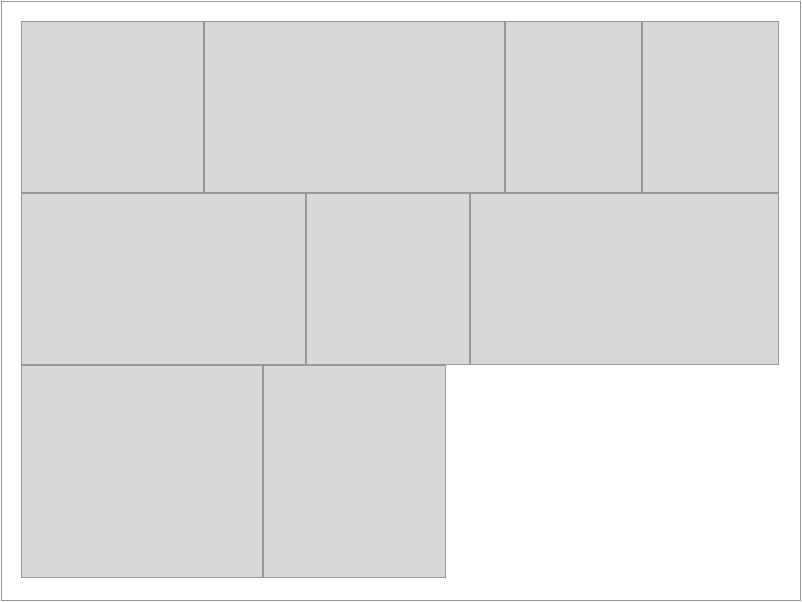# 木桶布局 原理与实现1、设置行高、行宽、边距
2、获取图片高、宽
3、将每个图片高度设置为行高，并等比例缩放图片宽度
4、遍历图片尺寸数组，从第一个图片开始，累计图片宽度，并将图片放入一个数组，当加上第n+1个图片的宽度后，累计宽度与行宽的差大于行高，从第n+1个图片开始重新累计图片宽度，并重新第n+1个开始放入新的数组中，以此获取多个包含着若干图片宽度之和与行宽相近的数组，将它们整成二维数组以便下一步处理。
5、将预处理好的二维数组，再次遍历，先计算图片处理后的宽度之width和与行宽row_width之比ratio，再用行高row_height与该ratio相除，获取等比例width等比例缩小成row_width得到的图片高度height，这个height就是图片实际应该设置的高，接下来按height通过等比例缩放来获取图片实际应该设置的宽度img_width，并更新原数组中的宽度。
6、处理后的数组就差不多是可实现木桶布局的图片尺寸了，但由于之前的计算中，由于可能浮点数运算然后四舍五入导致出现误差，所有还需要矫正一下，累计每一个图片组的宽度之和，计算与行宽的误差over_width，然后通过为每组最后一个图片增减over_width，矫正误差。

``````......
//artimages 是图片数组
foreach(\$artimages as \$k=>\$artimage){
if(!empty(\$artimage['production'])){
\$tmp['url'] = \$artimage['url'];
\$tmp['id'] = \$artimage['id'];
list(\$tmp['w'],\$tmp['h'])=getimagesize(storage_path('app').'/'.\$artimage['path']);
array_push(\$image_arr,\$tmp);
}
}

foreach(\$image_arr as \$k=>\$image){
\$image_arr[\$k]['w'] = intval(\$image['w'] * (300/number_format(\$image['h'],2,'.','')));
\$image_arr[\$k]['h'] = intval(\$row_height);
}

\$result = [];
\$tmp_width = 0;
\$i = 0;
foreach(\$image_arr as \$k=>\$image){
if(\$row_width < (\$tmp_width + \$image['w'] + \$space_width)){
if(abs(\$row_width - (\$tmp_width + \$image['w'] + \$space_width)) > \$row_height){

\$count = count(\$result[\$i]);//一组图片数量
//当前组图片累计的宽度 与 预设行宽 之比
\$ratio = number_format((\$tmp_width-\$count*\$space_width)/(\$row_width-(\$count+1)*\$space_width),2,'.','');

/*
* 调整该组图片应设行高
*/
\$tmp_height = intval(300 / \$ratio);
\$width = 0;
foreach( \$result[\$i] as \$index => \$val){
\$result[\$i][\$index]['h'] = \$tmp_height;
\$result[\$i][\$index]['w'] = intval(\$val['w'] * (\$tmp_height / \$val['h']));
\$width += \$result[\$i][\$index]['w'] + \$space_width;
}

\$over_width = \$row_width - intval(\$width + \$space_width);//当前行宽误差
\$result[\$i][\$index]['w'] += \$over_width;//消除误差
\$tmp_width = 0;
\$i ++;//接下来的图放入下行
}
}
\$result[\$i][] = \$image;
\$tmp_width += \$image['w'] + \$space_width;//记录新宽度

}
return \$result;``````